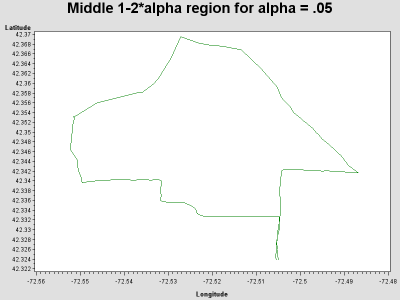Want to share your content on R-bloggers? click here if you have a blog, or here if you don't.The iPhone app Cyclemeter uses the phone’s GPS capability to record location and other data, and infer speed, while you ride. I took a ride near my house recently, and downloaded the data. I’d like to examine my route and my speed. A simple plot of the route is trivial in either SAS or R, but adding the speed data requires a little work. You can download my data from here and I read the data directly via URL in the following code.

SAS

In SAS, I first use proc import with the url filetype, as shown in section 1.1.6. I can then make a simple plot of the route using the i=j option to the symbol statement (as in section 1.13.5), which simply joins successive points.

`filename bike url 'http://www.kenkleinman.net/files/cycle-data-10022010.csv';proc import datafile=bike out=ride dbms=dlm;  delimiter=',';  getnames=yes;run;symbol1 i=j;proc gplot data=ride;  plot latitude * longitude;run;`I didn’t project the data, so this looks a little compressed north-south.

To show my speed at each point, I decided to make a thicker line when I’m going faster. To do this, I use the annotate macros discussed in section 5.2. I decided to use the %line macro to do this, but that requires each observation in the data set have a starting point and an ending point for its section of line. I use the lag function (section 1.4.17) in a separate data step to add the previous point to each observation. Then I create the annotate data set. Finally, I use the value = none option to the symbol statement to make an empty plot and the annotate data set draws the line for me.

`data twopoints;set ride;  lastlat = lag(latitude);  lastlong = lag(longitude);  if _n_ ne 1;run;%annomac;data annoride;set twopoints;%system(2,2,6);%line(longitude,latitude,lastlong,lastlat,  black,1,speed__miles_h_);run;symbol1 v=none;proc gplot data=ride;  plot latitude * longitude / annotate=annoride;;run;quit;`

The resulting plot shown below closely resembles the R plot shown at the top of this entry.R

In R, it’s as trivial to make the simple plot as in SAS. Just read in the CSV data from the URL (section 1.1.2, 1.1.6) make an empty plot (5.1.1), and add the lines (5.2.1).

`myride=read.csv("http://www.kenkleinman.net/files/cycle-data-10022010.csv")attach(myride)plot(Longitude, Latitude, type="n")lines(Longitude, Latitude)`Now I want to show the speed, as above. The lines() function has a lwd= option, but unfortunately, it’s not vectorized. In other words, it accepts only a scalar that applies to all the line segments drawn in a given call. To get around that, I’ll write my own vectorized version of lines() using the disfavored for() function. It calls lines() for each pair of points, with an appropriate lwd value.

`veclines = function(x, y, z) {  for (i in 1:(length(x)-1)) {    lines(x[i:(i+1)], y[i:(i+1)], lwd=z[i])  }}veclines(Longitude, Latitude, Speed..miles.h./2)`

The result is displayed at the top of this blog entry. In the next entry we’ll add more information to help explain why the speed varies.